# 一、二阶系统数学模型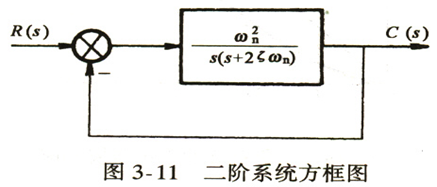ζ \ ζ ——阻尼比
ω n \ ω_n ——无阻尼自然振荡角频率
σ = ζ ω n \ σ=ζω_n ——衰减系数
ω d = ω n √ ( 1 − ζ 2 ) \ ω_d=ω_n √(1-ζ^2 ) ——系统的阻尼振荡角频率

# 二、二阶系统单位阶跃响应

## 1.   ζ = 0 \ ζ=0 (零阻尼)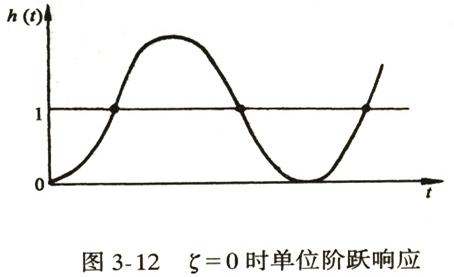## 2.   ζ > 1 \ ζ>1 ( 过阻尼)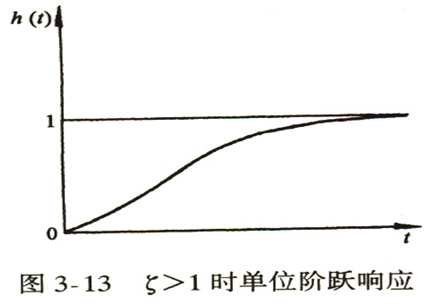## 3.   ζ = 1 \ ζ=1 ( 临界阻尼)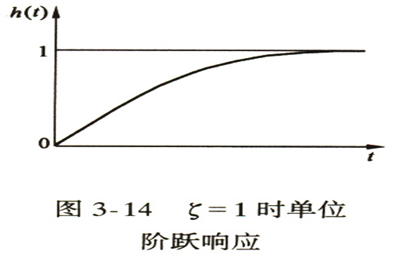## 4.   0 < ζ < 1 \ 0<ζ<1 ( 欠阻尼)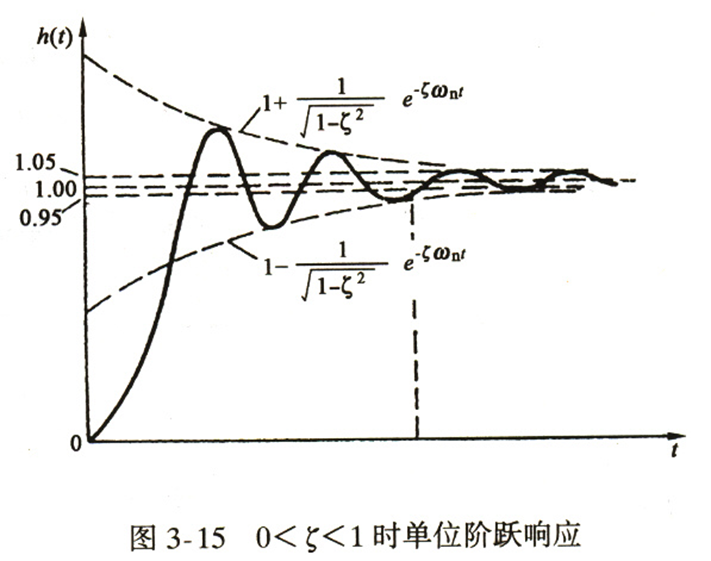## 5.总结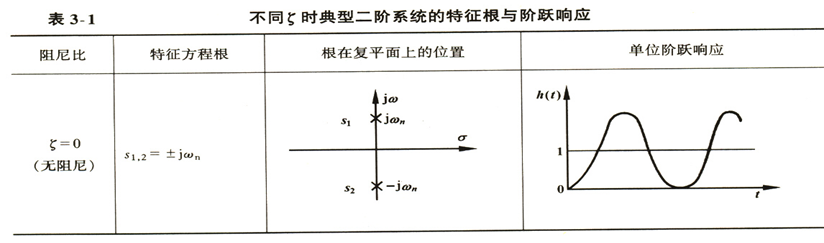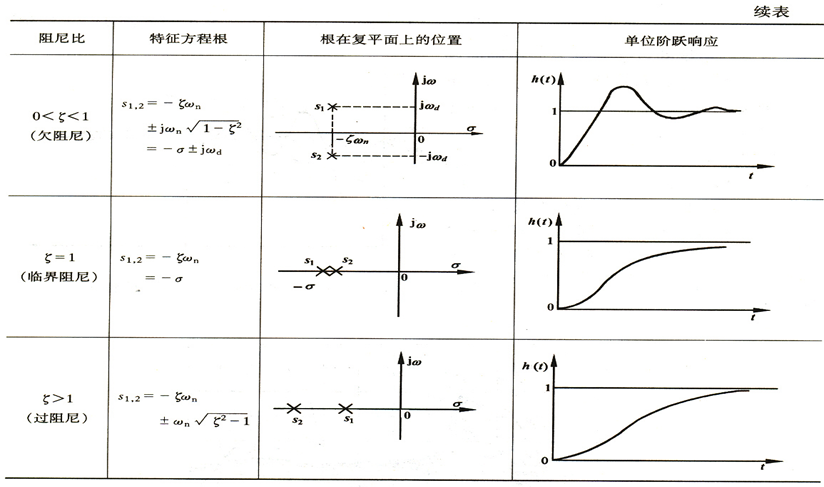# 三 、欠阻尼二阶系统性能指标计算

## 1.上升时间   t r \ t_r

t r = π − β ω n √ ( 1 − ζ 2 ) \ t_r=\frac{π-β}{ω_n √(1-ζ^2 )}

## 2.峰值时间   t p \ t_p

t p = π ω n √ ( 1 − ζ 2 ) \ t_p=\frac{π}{ω_n √(1-ζ^2 )}

## 3.超调量   σ % \ σ\%

σ % = e − ζ π √ ( 1 − ζ 2 ) \ σ\%=e^{\frac{-ζπ}{√(1-ζ^2 )}}

## 4.调节时间   t s \ t_s

t s = 4 ζ ω n \ t_s=\frac{4}{ζω_n } (   Δ = 2 % \ Δ=2\% )
t s = 3 ζ ω n \ t_s=\frac{3}{ζω_n } (   Δ = 5 % \ Δ=5\% )

## 5.延迟时间   t d \ t_d

0 < ζ < 1 \ 0<ζ<1 时，   t d = 1 + 0.7 ζ ω n \ t_d=\frac{1+0.7ζ}{ω_n }

# 四 、具有零点的二阶系统分析

1、原系统条件不变时，附加一个闭环零点，将使二阶系统阶跃响应的超调量增大，上升时间和峰值时间减小
2、零点距离极点越近，上述影响越显著
3、零点距离虚轴很远时，零点影响可忽略

11-15
12-181万+
11-272205
03-251万+
01-18
08-24117
08-101021
02-229153
05-062万+
07-124066
11-03
11-301万+
03-197384
04-264573
01-194998
01-142301

### “相关推荐”对你有帮助么？

•非常没帮助
•没帮助
•一般
•有帮助
•非常有帮助

©️2022 CSDN 皮肤主题：大白 设计师：CSDN官方博客被折叠的  条评论 为什么被折叠?到【灌水乐园】发言wang7301

¥2 ¥4 ¥6 ¥10 ¥20余额支付 (余额：-- )扫码支付获取中扫码支付点击重新获取扫码支付1.余额是钱包充值的虚拟货币，按照1:1的比例进行支付金额的抵扣。
2.余额无法直接购买下载，可以购买VIP、C币套餐、付费专栏及课程。余额充值